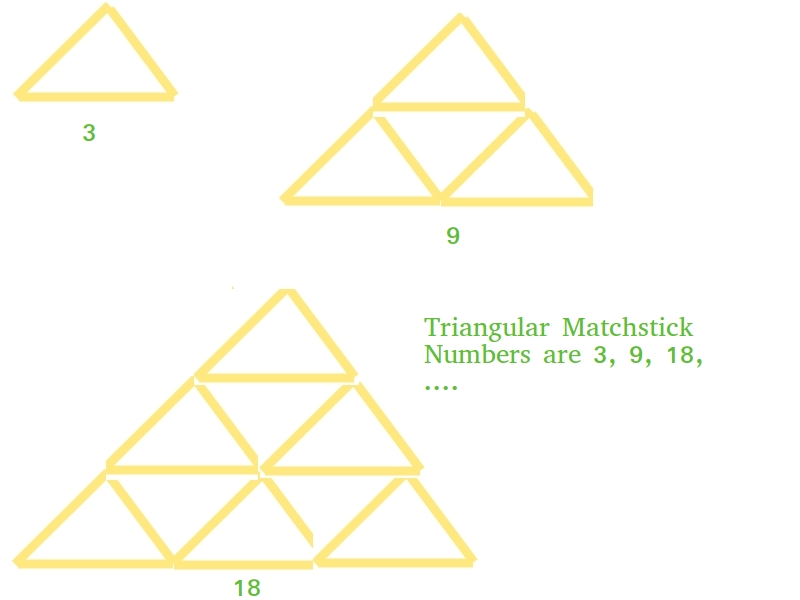Open in App
Not now

# Triangular Matchstick Number

• Difficulty Level : Easy
• Last Updated : 22 Jun, 2022

Given a number X which represents the floor of a matchstick pyramid, write a program to print the total number of matchstick required to form pyramid of matchsticks of x floors.

Examples:

```Input : X = 1
Output : 3

Input : X = 2
Output : 9 ```

This is mainly an extension of triangular numbers. For a number X, the matchstick required will be three times of X-th triangular numbers, i.e., (3*X*(X+1))/2## C++

 `// C++ program to find X-th triangular``// matchstick number` `#include ``using` `namespace` `std;` `int` `numberOfSticks(``int` `x)``{``    ``return` `(3 * x * (x + 1)) / 2;``}` `int` `main()``{``    ``cout<

## Java

 `// Java program to find X-th triangular``// matchstick number``public` `class` `TriangularPyramidNumber {``    ``public` `static` `int` `numberOfSticks(``int` `x)``    ``{``        ``return` `(``3` `* x * (x + ``1``)) / ``2``;``    ``}``    ``public` `static` `void` `main(String[] args)``    ``{``        ``System.out.println(numberOfSticks(``7``));``    ``}``}`

## Python3

 `# Python program to find X-th triangular``# matchstick number` `def` `numberOfSticks(x):``    ``return` `(``3` `*` `x ``*` `(x ``+` `1``)) ``/` `2``    ` `# main()``print``(``int``(numberOfSticks(``7``)))`

## C#

 `// C# program to find X-th triangular``// matchstick number``using` `System;` `class` `GFG``{``    ``// Function to ind missing number``    ``static` `int` `numberOfSticks(``int` `x)``    ``{``        ``return` `(3 * x * (x + 1)) / 2;``    ``}` `    ``public` `static` `void` `Main()``    ``{``        ``Console.Write(numberOfSticks(7));``    ``}``}` `// This code is contributed by _omg`

## PHP

 ``

## Javascript

 ``

Output:

`84`

Time Complexity: O(1)

Auxiliary Space: O(1)

My Personal Notes arrow_drop_up